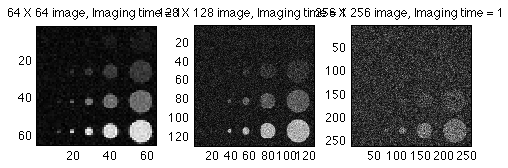## MATLAB-based demonstrations of signal and image behavior in MRI

Prepared for the 2014 UCLA/Semel Advanced Neuroimaging Summer Program

For a very gentle introduction to MATLAB, have a look at A short intro to MATLAB.Learn about plotting, Fourier transforms and convolution.

Contrast to Noise Ratio
Simulation of the effects of imaging time and matrix size on the visibility of objects of various size in MR imaging. Shows that smaller voxels does not necessarily mean better resolution.MRI contrast
Understanding the effects of sequence parameters on image contrast.K-Space Demos
Demonstration of important Fourier imaging effects including:
• MRI Raw Data
• What k-space data typically look like
• Resolution and k-space
• High and low spatial frequency information
• Smoothing and Sharpening
• Spike Artifacts
• Saturation
• Apodization
• Fourier shift theorem
• Motion artifact